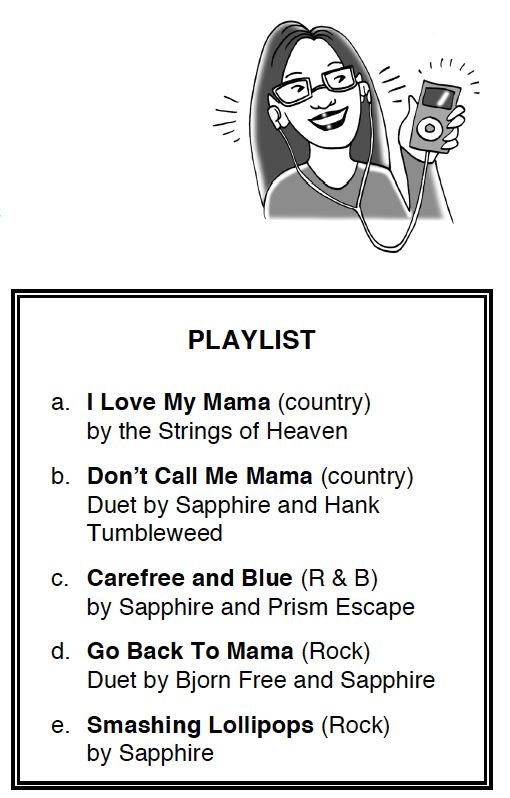Home > INT2 > Chapter 2 > Lesson 2.1.4 > Problem2-45

2-45.While waiting for a bus after school, Renae programmed her MP3 player to randomly play two songs from her playlist, at right. Assume that the MP3 player will not play the same song twice. Homework Help ✎

1. A sample space is a list of all possible outcomes for a probabilistic situation. List the sample space for all the combinations of two songs that Renae could select. The order in which she hears the songs does not matter for your list. How can you be sure that you listed all the song combinations?

Start with a, write out each possible pair starting with this letter. Then write out each new combination with b, and so on. Keep in mind there should be 10 pairs total.

There are 10 combinations: a & b, a & c, a & d, a & e, b & c, b & d, b & e, c & d, c & e, d & e.

2. Are each of the combinations of two songs equally likely? Why is that important?

See the Math Notes box in Lesson 2.1.4.

3. Calculate the probability that Renae will listen to two songs with the name “Mama” in the title.

Find all of the combinations with the word "Mama."

$\frac{3}{10}$

4. What is the probability that at least one of the songs will have the name “Mama” in the title?

If one element of the combination contains the word "Mama," then it applies to the question above.

$\frac{9}{10}$

5. Why does it make sense that the probability in part (d) is higher than the probability in part (c)?